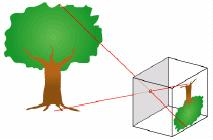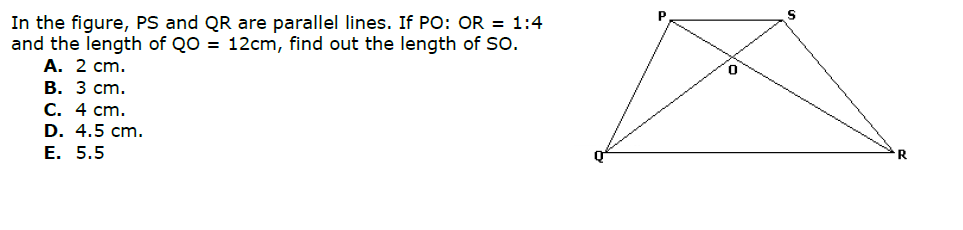# Similar Triangles

The current article deals with a frequently occurring topic in geometry in CAT- similarity of triangles. Most of you will notice that this topic is coming year after year in CAT and, as geometry questions in CAT are easy compared with other topics, mastering the concept is easier than mastering concepts in Algebra, Permutation, and Combination, or Probability.### Practice Problems

1.2.3. In ΔABC, right angled at B, BD is perpendicular to AC. Find the length of BD if AD and CD are
3 and 12 respectively.

## More Geometry Gyan :

• What is the solution?

By Akhilesh Poddar, 4 weeks ago

• In the island of Hoola Boola Moola , inhabitants have a...

By Sanket211, 3 months ago

• For a=0.9086, x= 1.0286 and a= -1.6627, x= 4.9173

By Aditya Dang, 4 months ago

• Since, x+1 satisfies the equation ax^2+ bx+ c=0. Theref...

By Aditya Dang, 4 months ago

• The HCF of 141 and 517 is 47. But 47 does not completel...

By Aditya Dang, 4 months ago

• 1. How many integral pairs (x, y), where 0< x, y <...

By lastknight99, 4 months ago

• Hi, It is given that a= (212)b . Forming an equation...

By Aditya Dang, 4 months ago

• Perimeter of circle is 2pi(793)^1/2.

By Aditya Dang, 4 months ago

• Area of ΔDOC is 21cm^2.

By Aditya Dang, 4 months ago

• Length of smaller of two sides is equal to 36 units.

By Aditya Dang, 4 months ago

• Length of AB is (17)^1/2 units.

By Aditya Dang, 4 months ago

• The sum borrowed is Rs. 3300.

By Aditya Dang, 4 months ago